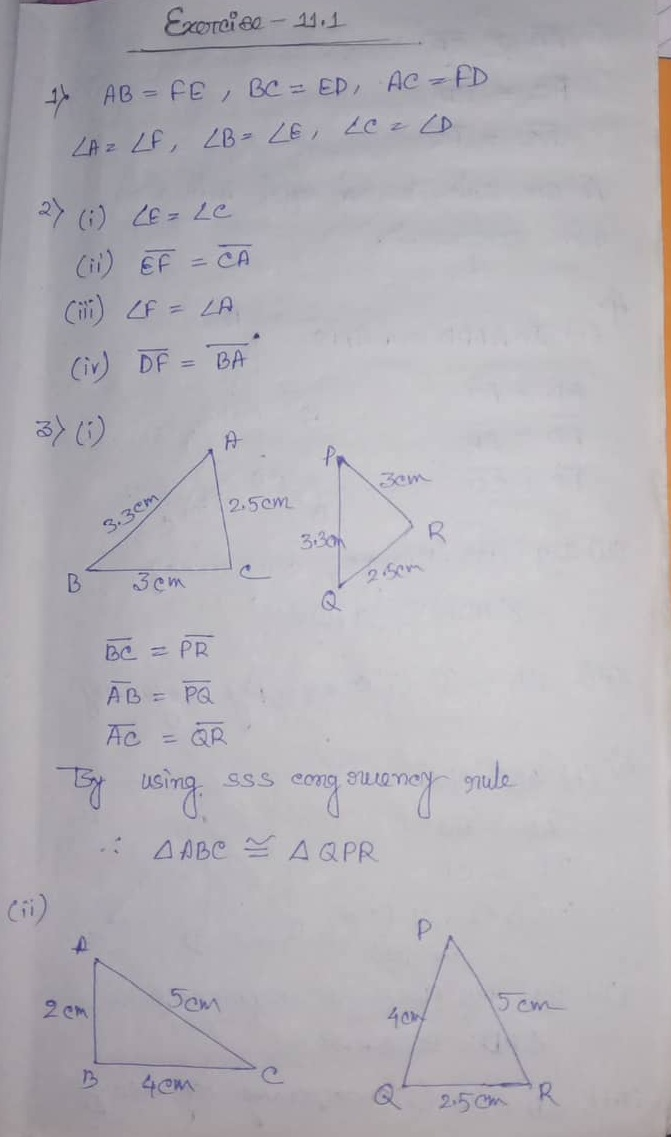# ML Aggarwal CBSE Solutions Class 7 Math Eleventh Chapter Congruence of Triangle Exercise 11.1

### ML Aggarwal CBSE Solutions Class 7 Math 11th Chapter Congruence of Triangle Exercise 11.1

(1) If ΔABC and ΔDEF are congruent under the corresponding ABC <–>FED, write all the corresponding congruent parts of the triangles.
(2) if ΔDEF ≅ ΔBCA, then write the part(s) of ΔBCA that corrospond to
(i) ∠E
(ii)
__
EF
(iii) ∠F
(iv)
__
DF

(3) In the figures given below, the lengths of the sides of the triangle are indicated. By using SS congruency rule, state which pairs of triangles are congruent. In case of congruent triangles, write the result in symbiotic form:

Solution:(4) In the adjoining figure, Ab = 5 cm, AC = 5 cm, BD = 2.5 cm and CD = 2.5 cm.
(i) State the three pairs of equal parts in ΔADB and ΔADC.
(ii) Is ∠B = ∠C? Why?

Solution:(6) In the figures given below, the measures of some parts of the triangles are indicated. By using SAS rule of congruency, state which pairs of triangles are congruent. In case of congruent triangles, write the result in symbolic form.

Solution:(7) By applying SAS congruence rule, you want to establish that △PQR ≅ △FED. It is given that PQ = FE and RP = DF. What additional information is needed to establish the congruence?

Solution: ∠P = ∠F

(8) You want to show that ΔART ≅ ΔPEN
(a) If you have to use SSS criterion, then you need to show
(i) AR =
(ii) RT =
(iii) AT =
(b) If it is given that ∠T = ∠N and you are to use SAS criterion, you need to have
(i) RT = and (ii) PN =

(9) You have to show that △AMP ≅ △AMQ.
In the following proof, supply the missing reasons.

 Steps Reasons (i) PM = QM (i) …. (ii) ∠PMA = ∠QMA (ii) …. (iii) AM = AM (iii) …. (iv) △AMP ≅ △AMQ (iv) ….

(i) State three pairs equal parts in △PSR and △RQP? Give reasons.
(ii) Is △PSR ≅ △RQP? Give reasons.
(iii) Is PS = RQ? Why?
(iv) Is ∠S = ∠Q? Why?

Solution:(11) In the adjoining figure, AB = DC and ∠ABC = ∠DCB.
(i) State three pairs of equal parts in △ABC ≅ △DCB? Give reasons.
(ii) Is △ABC ≅ △DCB? Give reasons.
(iii) Is AC = DB? Why?

(i) State three pairs of equal parts in △ABC and △ABD.
(ii) Is △ABC ≅ △ABD? Give reasons.
(iii) Is BC = BD? Why?
(iv) is ∠C = ∠D? Why?

Solution:Updated: November 11, 2019 — 1:55 pm